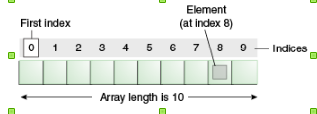# How to declare and initialize an array in Java

An array is a container object that holds a fixed number of values of a single type. The length of an array is established when the array is created. After creation, its length is fixed.

Each item in an array is called an element, and each element is accessed by its numerical index. As shown in the preceding illustration, numbering begins with 0. The 9th element, for example, would therefore be accessed at index 8.1) Single dimensional array declaration

```int[] array1 = new int;
int[] array2 = {1,2,3,4,5};
int[] array3 = new int[]{1,2,3,4,5};```

### 2) Multidimensional array declaration

`int[][] array4 = new int;`

### Java Array Declaration Example

You can use Arrays.toString(array) to print the content of the single dimensional array and Arrays.deepToString(array) to print the multi dimensional arrays

```import java.io.IOException;
import java.util.Arrays;

public class Main {

public static void main(String[] args) throws IOException {

//How to declare and initialize an array in Java
int[] array1 = new int;
int[] array2 = {1,2,3,4,5};
int[] array3 = new int[]{1,2,3,4,5};

//Multidimensional array declaration
int[][] array4 = new int;

System.out.println(Arrays.toString(array1));
System.out.println(Arrays.toString(array2));
System.out.println(Arrays.toString(array3));
System.out.println(Arrays.deepToString(array4));

}
}```

Output

```[0, 0, 0, 0, 0]
[1, 2, 3, 4, 5]
[1, 2, 3, 4, 5]
[[0, 0, 0], [0, 0, 0]]```